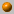# Computational Geometry Fall 2005/6

Instructor: Dan Halperin , danha AT post
Teaching Assistant: Eitan Yaffe, eitanyaf AT post

Wednesday, 16:00--19:00
Schreiber 006

Office hours: Wednesday, 19:00--20:00
Schreiber 219

The course covers fundamental algorithms for solving geometric problems such as computing convex hulls, intersection of line segments, Voronoi diagrams, polygon triangulation, and linear programming in low dimensional space. We will also discuss several applications of geometric algorithms to solving problems in robotics, GIS (geographic information systems), computer graphics, and more.

### Bibliography:

The main textbook of the course is
Computational Geometry: Algorithms and Applications (CGAA), 2nd edition
by M. de Berg, M. van Kreveld, M. Overmars, and O. Schwarzkopf.

A bibliographic list for the course.

The final grade will be determined by: 90% - a final exam, and 10% - homework assignments.

### Course Summary:

Below you'll find a very brief summary of what was covered in class during the semester. This should not be taken as a complete description of the course's contents.

For an outline of the course, see for example, the course summary in the 2004 computational geometry course .7.11.05 Introduction
What is Computational Geometry? Course outline, motivation and techniques. Naive convex hull algorithms (see Chapter 3 in O'Rourke's book). Orientation (Side-of-line) test.

Video segments:Searching with an Autonomous Robot
by Sándor P. Fekete, Rolf Klein, and Andreas Nüchter
SoCG 2004, video proceedings, compgeom.poly.edu/acmvideos/socg04video/Exact Minkowski Sums of Convex Polyhedra
by Efi Fogel and Dan Halperin
SoCG 2005, video proceedings, compgeom.poly.edu/acmvideos/socg05video/index.html9.11.05 Convex hull computation continued; Line segment intersection

Gift wrapping. Graham's O(n log n) algorithm (Chapter 1 in CGAA). Lower bound for convex hull algorithms.

Output sensitive algorithm to compute the intersections of line segments: Bentley Ottmann's plane sweep (Chapter 2 in CGAA).16.11.05 Sweep line; DCEL; Map overlay; Douglas-Peuker polyline simplefication

The doubly-connected edge list (DCEL), and overlay of planar subdivisions (CGAA, Chapter 2).

The Douglas-Peuker algorithm for line simplification. Video segment no.~1 in CG Video Review 1994, An O(n\log n) implementation of the Douglas-Peucker algorithm for line simplification, by Hershberger and Snoeyink. For details see Proc. of the 10th ACM Symposium On Computational geometry, pp. 383--384.

The last segment in CG Video Review 1992, by Tal, Chazelle, and Dobkin. Based on the paper: An optimal algorithm for intersecting line segments in the plane, by Chazelle and Edelsbrunner, J. of the ACM, Vol. 39, 1992, pp. 1--54. For recent advances on this problem see "Notes and comments" at the end of Chapter 2 of CGAA.23.11.05 Polygon triangulation; Art gallery problems

Introduction to triangulation of polygons.

We have shown that \$\lfloor n/3 \rfloor\$ guards are sometimes necessary and always sufficient to guard a simple polygon with n vertices. We have shown that every simple polygon can be triangulated; that the triangulation graph is 3-colorable; that the dual of the triangulation graph is a tree.

A naive algorithm that mimics the triangulation existence proof can triangulate a simple polygon with n vertices in O(n^2) time. A more efficient algorithm does it in O(n\log n) by first decomposing a polygon into monotone polygons and then triangulating each of the resulting monotone subpolygons.

The material covered in class could be found in O'Rourke's book, Chapters 1 and 2, and in CGAA, Chapter 3. (The description of how to decompose a polygon into y-monotone polygons follows O'Rourke's presentation.)

Guest talk: Jur van den Berg from Utrecht University on a variety of porblems in robot motion planning and automation.30.11.05 Tetrahedralization; Intersection of halfplanes; Casting

We completed the triagulation algorithm. Next, a few facts about tetrahedralization where surveyed: not every polyhedron is tetrahedralizable with vertices of the polyhedron only (see also Assignment no. 2); in general putting guards at the vertices of a polyhedron is insufficient for guarding the polyhedron; there are polyhedra with \$n\$ vertices that require \$\Omega(n^{3/2}))\$ guards.

We have shown an \$O(n\log n)\$ algorithm for computing the intersection of \$n\$ halfplanes. This question was motivated by a problem in casting: computing whether a given polyhedron is castable. See Chapter~4 in CGAA.7.12.05 Linear Programming

We presented the linear programming problem, then a randomized incremental algorithm for LP in 2D, running in expected linear time, and finally "backward analysis" for randomized algorithms. See Chapter 4 of CGAA. Randomized linear time algorithm for finding the minimum enclosing disc of a set of points in the plane. Sketch of LP in 3D. CGAA, Chapter 4.14.12.05 Linear Programming cont'd; Orthogonal range search

Linear time LP by Meggido, from Preparata-Shamos, Section 7.2.5.

Orthogonal range search I: kd-tress. CGAA, Chapter 5.21.12.05 Orthogonal range-search cont'd; introduction to Point Location

Range tree, fractional cascading. CGAA, Chapter 5.

Trapezoidal decomposition of planar maps revisited. Simple (but inefficient) solutions to the point location problem28.12.05 Point location; introduction to Voronoi diagrams

History over trapezoidal map strcuture for point location in expected logarithmic time. Relaxing the general position assumption. CGAA, Chapter 6.

What are Voronoi diagrams. Basic properties of the standard (L_2) diagram of point sites in the plane. CGAA, Chapter 7.4.1.06 Voronoi diagrams

Further properties of the standard diagram of point sites in the plane. Fortune's sweep algotihm for computing the diagram in time O(n\log n). CGAA, Chapter 7.

Video segments: (i) "Visualizing Fortune's sweepline algorithm" by Seth Teller and (ii) "Moving a disc between polygons" by Stefan Schirra (both from the ACM CG Video Review 1993).11.1.06 Arrangements of lines; duality

Point-line duality. Incremental construction of arrangements of lines. CGAA, Chapter 8.

Computing the smallest area triangle determined by a set of points. Edelsbrunner's book, Section 12.4.18.1.06 Discrepancy; introduction to triangulations; overveiw of CGAL

Halfplane discrepancy. CGAA, Chapter 8.

Introduction to tringulation of point sets.

Guest talk by Efi Fogel; The hitchhiker's guide to CGAL.25.1.06 Delaunay triangulation

Legal triangulations, Delaunay triangulations, RIC of Delaunay. CGAA Chapter 9.